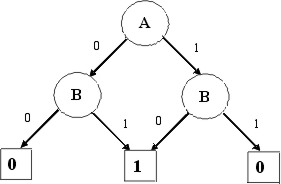# BDDs, OBDDs and ROBDDs

Given that Randy Bryant has just been awarded the Kaufmann prize for his work on formal verification, I thought it may be appropriate to talk a little more about some of his work and in particular his seminal work on binary decision diagrams or BDDs. Now I could just point you to his paper, or to many of the other academic works on this subject, but I wont because that all get very quickly into formal descriptions of Boolean algebra that are not really that helpful to understanding what these things are. So lets just keep it simple.

It all starts with a simple if-then-else operator that is applied to the variables in a system. So if we consider a simple system with one Boolean variable, then it can take the value of 0 and 1. Now if we have a system with two variables, then we can start to draw a diagram that shows the outcomes of each variable taking on certain values. Take a look at the BDD shown below.Here we see that we first test A. If it is a 0 we follow the left branch and test B. If B is a 0 then the result is a 0, otherwise the result is 1. But is A were 1 then when we test for B we get an result of 1 if B was 0 and 0 otherwise. In other words we have a Boolean XOR function.

Now consider the expression X = (a == b) or (c == d). We can draw the full tree as shown below.The first thing that we notice about this tree is that it is getting big, even for a simple expression. The second thing you will not is that there is a lot of repetition in the graph and some redundant tests, such as those in the middle of the graph where the outcome has already been decided. So we can start to collapse the tree to get rid of them. As a result, the tree now looks like this.Now there is only one group of the c == d test and that test is bypassed if the a == b test fails. The other thing that you will note is the order in which we made the tests, a then b then c and finally d. This may not have been the best order in which to do them, but in this case it turns out to be quite good. Equally good would have been c,d,a,b or c,d,b,a. What would not have been good would have been a,c,b,d. So this is what is meant by ordering, and what we have now created is a reduced, ordered BDD or ROBDD for short.

And that in a nutshell is what it is all about. Now that we have a compact graph for the system, we can start to reason about it and that is what formal verification tool do. Now I should also say that is just one of the techniques that formal tools use, as well as equivalence checkers and logic synthesis tools.

Brian Bailey – keeping you covered

[amazon_enhanced asin=”1441950478″ /] [amazon_enhanced asin=”0792396529″ /] [amazon_enhanced asin=”0898714583″ /] [amazon_enhanced asin=”0387500340″ /]